# Quan Attenuator

New for May 2009! And still under construction! Clifton Quan's attenuator uses the unequal split Wilkinson, a.k.a. the split-tee power divider. We found it by googling patents that reference Parad and Moynihan's equations. This one was tough to find, they misspelled "Moynihan" "Moyniham". One of the claims of the patent is that the value of attenuation is not a strong function of resistor tolerance, it is more a function of the impedance tolerance you can achieve. We'll examine that claim.

It is covered by US patent 7276989, in case you were thinking about using it!. It was issued on October 2, 2007, with Clifton Quan, Stephen M. Schiller, Yanmin Zhang listed as inventors. The patent is assigned to Raytheon. Cliff is like a modern Thomas Edison, he forty US patents!

The patent shows a few different ways to form an attenuator from unequal splitters. The simplest concept is shown in the schematic below. As you will see, for low and high values of attenuation, the required line impedances will be difficult to achieve; one solution (as the inventors point out) is to add a transformer section to the Port 1 side of the network.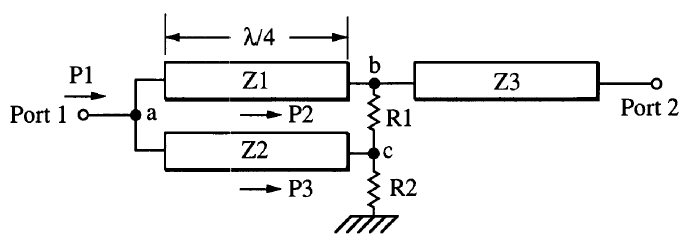Let's come up with some equations to pick values for the line impedances and resistors. First, we note that the attenuator is related to the unequal-split Wilkinson, shown below.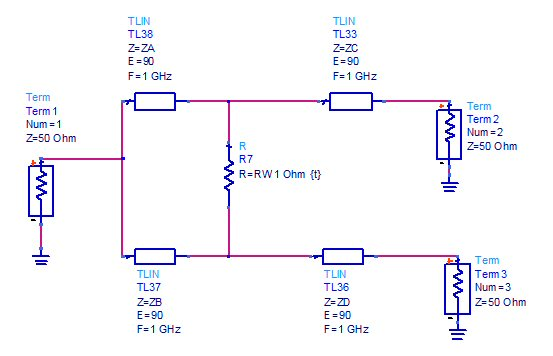Unequal-split Wilkinson schematic

The attenuator throws away one of the output transformers, and terminates that arm with a resistor. The corresponding schematic is shown below.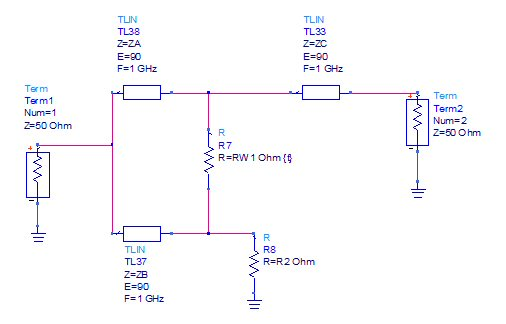Quan's attenuator schematic

If you've studied the topic of unequal split Wilkinson splitters here at Microwaves101, you know that there are many ways to pick the impedance and resistor values. Below we will develop equations based on Parad and Moynihan's Split-Tee Power Divider, as well as the "Choose ZA" method we have developed for this web site.

Looking at the power divider, we define the ratio of the two output powers as alpha: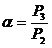Next we will define Att, which is the attenuation from port 1 to port 2: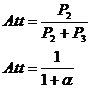Now we can resolve the "choose ZA" equations in terms of Att: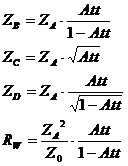Finally, we replace arm ZD with a termination R2 to arrive at the two-port Quan attenuator: network:

.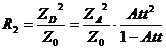You can discard the equation for ZD! Similarly, we came up with the solution that obeys the Split Tee equations (where you don't get to choose any of the impedances). Below we present both versions of the equations for the attenuator. On the left, we allow the user to choose ZA, on the right we use Parad and Moynihan's equations from Split Tee Power Divider. If you aren't used to Agilent's ADS software, the {t} means the quantity is tunable during a simulation.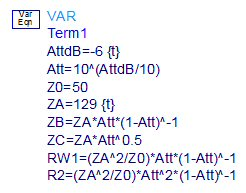"Choose ZA" equations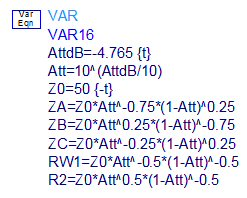Based on Split-Tee equations

### 1 dB attenuator example

For a 50 ohm, 1 dB attenuator, if we follow the Split Tee equations, we get the following response (and component values, if you can read them!)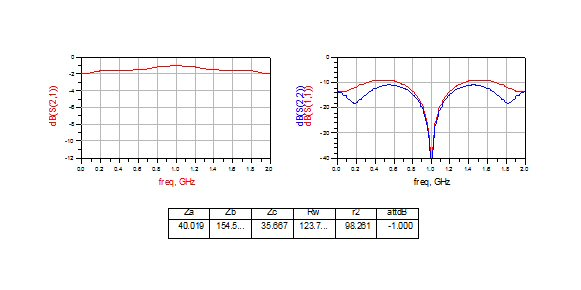By choosing ZA and optimizing the input and output match, we get the following result, which is much flatter attenuation and broader impedance match over frequency. Here ZA is 58 ohms, in the initial case it was ~40 ohms.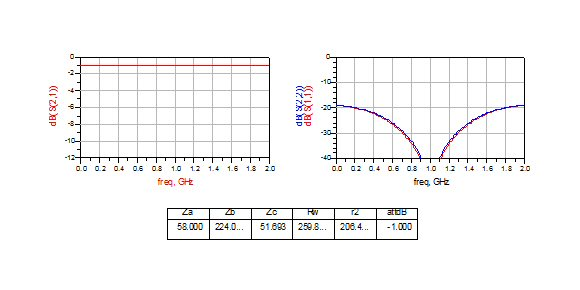### 3 dB example

Based on Split Tee equations, you get this result. Here ZA=~70.5 ohms.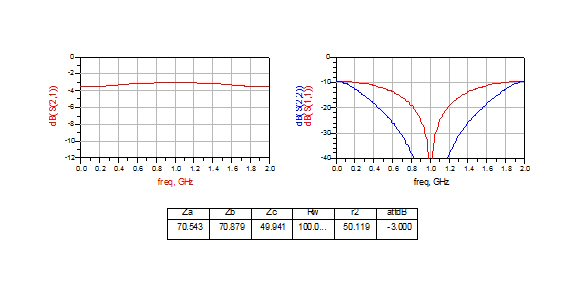By choosing ZA=79 ohms, you get a little more bandwidth, but not as much improvement as the 1 dB case. Notice a trend here?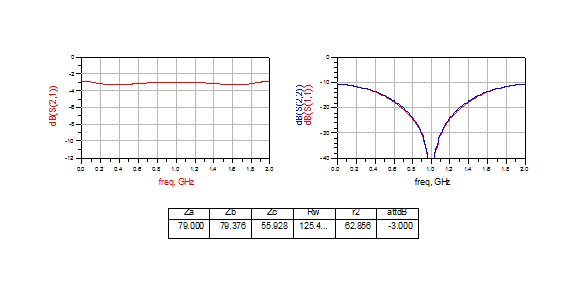### 6 dB example

In our final example, the difference between the Split Tee solution and the optimized solution is negligible. For the Split Tee response, ZA=~131 ohms.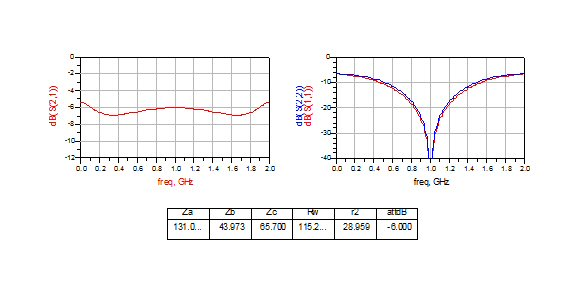In the optimized response, we lowered ZA to 121 ohms.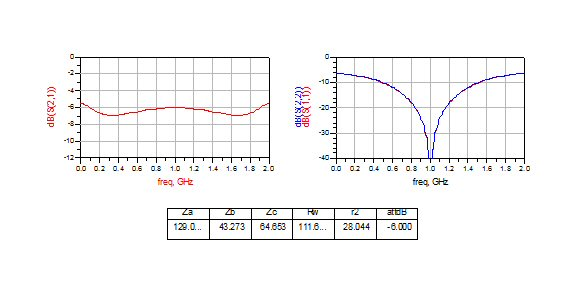In conclusion, it is probably best in this case not to rely on the split tee equations to give the best result.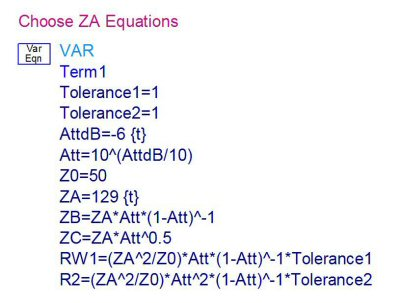Let's look at the invention's claims that inaccuracies in resistor values don't affect attenuation much. First, we add two more variables to the equations set. Tolerance1 changes the value of the dump resistor Rw, Tolerance2 chances the value of the terminating resistor R2. While we're on the subject, note that (ideally) all of attenuated power is dissipated in R2, so for power applications this better be a beefy component with good heat sinking!

Below is the nominal response for the 6 dB case, we added a marker so it's easier to see the attenuation value (S21).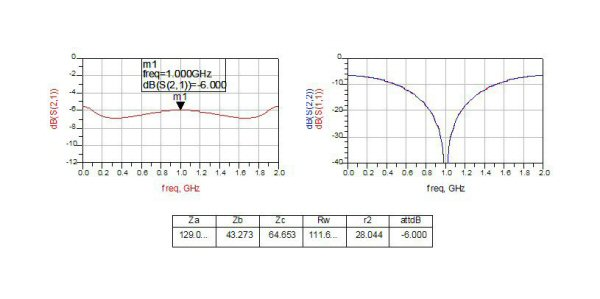We increased both resistors by 20% from ideal. The results show that attenuation only drifted by 0.081 dB.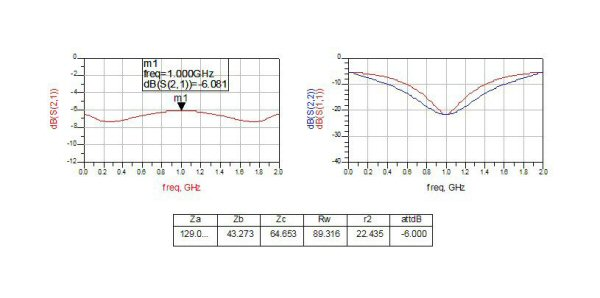Next decreased both resistors by 20% from ideal. Here, attenuation drifted by only 0.054 dB.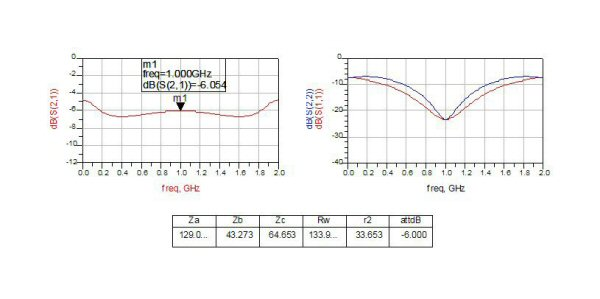Last, we increased Rw by 20%, and decreased R1 by 20%. In this case attenuation changed by just 0.065 dB.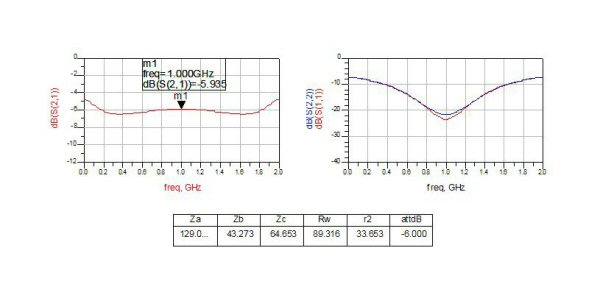In conclusion, the claim is well justified!

Author : Unknown Editor